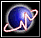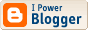Yet another weird SF fan
 I'm a mathematician, a libertarian, and a science-fiction fan. Common sense? What's that?Go to first entry

## Archives

<< current

 Yet another weird SF fan

### A Suggestion for the American Mathematical Society

As I've said before:

Please note that the {subequations} environment in $$\rm\LaTeX$$ will increment the main equation counter even when there are no equation numbers displayed. If you forget that, you might write an article in which the equation numbers skip from (17) to (20), causing the copy editors to wonder if you've discovered a new method of counting.
In view of the fact that authors frequently expect the {subequations} environment in $$\rm\LaTeX$$ to not increment the main equation counter when there are no equation numbers displayed, it might make sense to redefine {subequations} to fit their expectations. This can be done as follows:
\newenvironment{subequations}{%
\refstepcounter{equation}%
\protected@edef\theparentequation{\theequation}%
\setcounter{parentequation}{\value{equation}}%
\setcounter{equation}{0}%
\def\theequation{\theparentequation\alph{equation}}%
\ignorespaces
}{%
\ifnum\c@equation=0%
\setcounter{equation}{\value{parentequation}}%
\else
\setcounter{equation}{\value{parentequation}}%
\fi
\ignorespacesafterend
}

On the other hand, it may interfere with someone's workflow.Vader said...
 Profiles My Blogger ProfileX-treme Tracker The Atom Feed